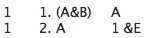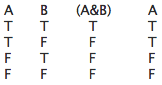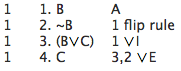# [SL16] Soundness

## §1. Metatheory

You have studied how to make derivations in our natural deduction system; you learned how to use the rules to show that a formula is derivable from other formulas. But now let us reflect on the strengths and weaknesses of this rule system. Instead of working within the system, you will study about the system.

This sort of study is called metalogic or metatheory, and is an important part of the study of logic. For any tool, one should learn not only how to use the tool, but one should learn about the tool-- what the tool can and cannot do. Here are some questions one might ask:

• Why are these rules good rules?
• Are some other rules better?
• What happens if we change the rules?
• Should we change the rules?

## §2. Two sound rules

One reason that the rules are good is that the rules ensure that each formula you write down is entailed by its dependencies. That is, for each line of a derivation, if the dependencies are true, then the formula is true.

Consider this short derivation:If the dependency of line 1 is true, then the formula on line 1 is true. Line 1 is "(A&B)". The dependency of line 1 is line 1, which is "(A&B)". And if "(A&B)" is true then "(A&B)" must be true:

(A&B) ⊨ (A&B)

In other words, "(A&B)" entails "(A&B)".
(Note that the symbol "⊨" is the double turnstile which you learned about in SL05, not the single turnstile "⊢" discussed here.)

If the dependency of line 2 is true, then the formula on line 2 is true. Line 2 is "A". The dependency of line 2 is line 1, which is "(A&B)". And, if "(A&B)" is true then "A" must be true:

(A&B) ⊨ A

In other words, "(A&B)" entails "A". You can see that from a truth table:This truth table shows that in every case where "(A&B)" is true, "A" is true too.

In a certain sense, you won't go wrong when you use Rule A or Rule &E. Rule A and Rule &E are both sound rules: when following these rules, the formula you write down is entailed by its dependencies.

Explain why &I, ∨I and ∨E are all sound rules.

## §3. The soundness of the system

If you think about each of the rules in our natural deduction system, you will see that each of the 12 rules is sound. (We won't discuss each of the rules here, but you can verify yourself that each is sound.) So at every line in every derivation, the formula on that line is entailed by its dependencies.

Our system, therefore, has the property of soundness: if a formula is derivable in the system from some formulas, it is entailed by the formulas.

More precisely, soundness means that:

For any formula φ, if ⊢ φ, then ⊨ φ,

and

For any formula φ, and list of formulas X, if X ⊢ φ then X ⊨ φ.

Would the system be sound if we add the following rule?

If you have derived (φ∨φ), you can write down φ, depending on everything (φ∨φ) depends on.

Would the system be sound if we remove rule &I?

Would the system be sound if we change rule →I this way? Why or why not?

If you have derived φ, and you have derived ψ, you can write down (φ→ψ), depending on everything ψ depends on except φ.

## §4. Why sound rules are good

At this point one might wonder why it is good to have a system that is sound. One way to think about that would be to suppose an unsound rule is added to the system:

Flip rule

If you have derived φ, you can write down ~φ, depending on everything φ depends on.

Then one could make the following derivation:Thus, C is derivable from B. And, by changing "B" to any formula φ and "C" to any formula ψ, one can show that φ is derivable from ψ. In the revised system, anything is derivable from anything!

The revised system is useless, insofar as one hopes to use a deduction system to show when a conclusion follows from some premises in an argument. For a conclusion of an argument does not always follow from the premises.

Suppose we add the flip rule and remove Rule ∨E from our natural deduction system.
Would the resulting system be sound?

## §5. Derivations and truth tables

One way to find out whether a sequent is valid is to make a truth table. For example, a truth table will show whether or not this is a valid sequent:

((P ∨ Q)→ R) ⊨ (P → R)

In the truth table, if there is a line where "((P ∨ Q)→ R)" is true and "(P → R)" is false, then the sequent is invalid. Otherwise the sequent is valid.

Since our natural deduction system is sound, you can use it to show that a sequent is valid. You can make a derivation to show the right hand side of the sequent is derivable from the left hand side:

((P ∨ Q)→ R) ⊢ (P → R)

and, then, since our system is sound, it follows that the sequent is valid.

((P ∨ Q)→ R) ⊨ (P → R).